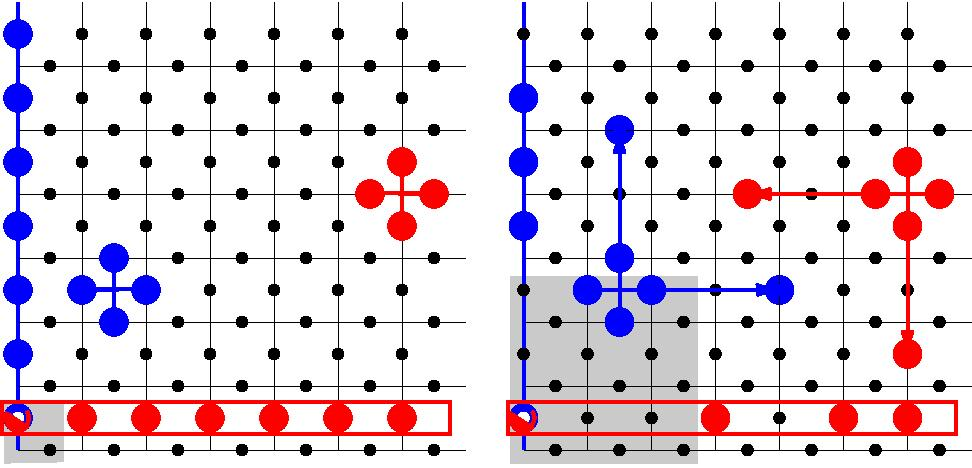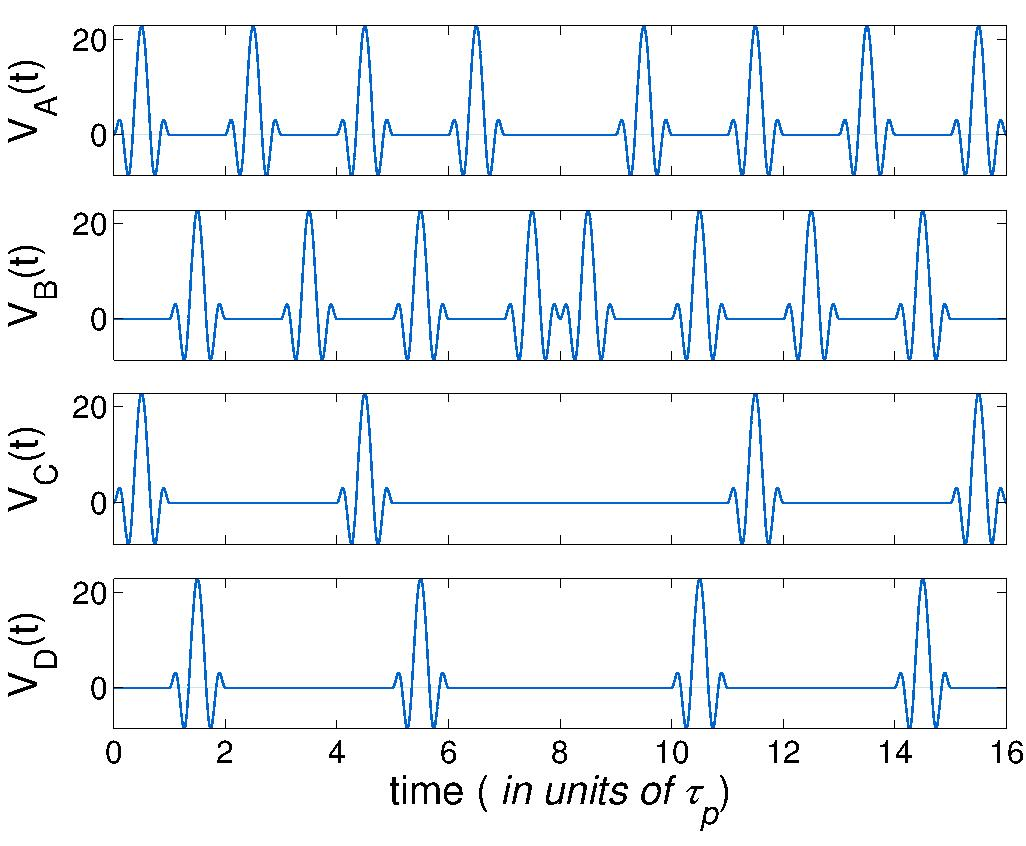# Current projects

## Quantum error correctionBack in 1996 when Peter Shor employed concatenated quantum codes to prove the existence of a finite fault-tolerant threshold to scalable quantum computation, he never intended his original construction to be used in an actual quantum computer. Fault-tolerant (FT) means capable of operating even with faulty hardware. Concatenated codes are equivalent to using multiple layers of encoding: a single qubit is encoded in, e.g., five, then each of those again in five, etc, with qubits at deeper levels seeing progressively weaker decoherence levels as long as the physical error rate is below certain threshold. Such a construction is fragile, cumbersome to operate, and the resulting threshold to scalable quantum computation is small, of order 10-4 error probability per elementary quantum gate. Also, concatenated codes encode very few qubits k per block of length n: asymptotically, these codes have zero rate R = k/n. Thus, to avoid infinite overhead, one has to operate at the error levels substantially below the threshold.

Only in mid 2000s concatenated codes have been supplemented with Kitaev's surface (toric) codes with simpler low depth structure and higher fault-tolerant (FT) threshold at around 1% [Fig. 1]. Surface and related codes have a provably unique advantage of requiring only local gates with a 2D qubit layout, but they also have zero asymptotic rates: One needs some 108 qubits to build a useful quantum computer (QC) based on such codes.

In the past few years, several large families of finite-rate quantum low-density parity check (LDPC) codes have been constructed. These codes share many of the benefits of the surface codes, with the necessary exception that non-local gates are required.

Paper : Known quantum LDPC codes have power-law distance scaling with block length, d=nα, with α<1, which guarantees that any error of size up to ⌊d/2⌋ can be corrected. Naively, one would expect such codes to be bad, since a typical error involves a number of qubits pn scaling linearly in n (here p is per-qubit error probability). In this paper we demonstrated this is not so. With LDPC codes where each stabilizer generator has a finite weight, and each qubit is involved in a finite number of stabilizer generators, at small p a typical error has a pattern of disconnected clusters each of which can be corrected independently. With the help of repeated measurements, this also works in the presence of syndrome measurement errors. Basic conclusion is that quantum LDPC codes have a finite fault-tolerant threshold. This is the only known class of codes that combine a finite rate and a finite fault-tolerant error correction threshold.

As argued recently by Daniel Gottesman, this could produce enormous savings in the number of qubits needed to build a useful quantum computer. Indeed, with a zero-rate code like the surface codes, each additional encoded qubit protected by the code requires a whole block of code to be included. For better protection, each code block has to be larger. But with a finite-rate code family, the fraction of encoded qubits remains finite. To increase the number of encoded qubits, one may need a longer code, but that also increases the quality of the code: the qubits also get better protected!

Paper : We use simple geometrical tricks to construct several new families of quantum LDPC codes with finite rates, improving on the parameters of known codes. Many of the resulting codes are quantum LDPC codes that satisfy the condition for fault-tolerance.

Paper : At small bit error probabilities p and with a good decoder, large enough quantum code can be used to correct nearly all errors with high probability, while at p exceeding certain threshold value pc, the probability of successful decoding falls down precipitously. We show that when maximum-likelihood decoding is used, the decoding threshold corresponds to an actual phase transition in certain non-local spin model associated with the code. The transition happens by proliferation of extended defects which generalize the notion of domain walls to non-local spin models. In quantum LDPC code families with finite rates the number of distinct classes of such extended defects is exponentially large, corresponding to extensive ground state entropy of these codes. Here, the transition can be driven by the entropy of the extended defects, a mechanism distinct from that in the local spin models where the number of defect types (domain walls) is always finite.This is cool since a quantum error correcting code can be used to construct a non-trivial non-local spin model. Also, locating a threshold by simulating a spin model is typically easier. Maximum-likelihood decoder is supposed to give the highest threshold. Thus, one can use this mapping to compare different code families irrespective of the decoder used, and to get an absolute measure of decoder performance.

## Dynamically-corrected gates

Over several years, we've been working on design and applications of the pulse-based control approach similar to dynamical decoupling (DD) used in nuclear magnetic resonance (NMR), see the section on quantum coherent control below. Basic advantage is that DD allows to suppress the effect of decoherence due to low-frequency environment modes without involving any additional qubits. In many cases, like the famous 1/f noise, this decoherence source is primarily responsible for making coherence times shorter. Thus, incorporating DD sequences into quantum coherent control protocol could shift some of the burden from quantum error correction, e.g., permitting a longer error correcting cycle.

Paper : An important recent result is the construction of a sequence of pulses implementing a universal set of quantum gates for a system of qubits with always-on Ising couplings forming a pattern of a sparse bipartite graph. The idea is to run two global decoupling sequences on the qubits from the two sublattices, changing the sequence on the pairs of qubits that need to be coupled (see Fig. 2). In this paper we present the pulse sequences and demonstrated simulations of repeated quantum error correction (Zeno cycle) using a chain of five qubits and the error-detecting code [[4,2,2]].

Paper : Here we present a detailed analysis of the errors associated with the gates constructed by us previously. Since the pulses are constructed using the perturbation theory to K=2nd order, the biggest errors are formed by three and more bonds. This implies correlations across clusters of four and more qubits. Nevertheless, careful analysis shows that it is possible to use this gate set to construct quantum memory using the toric code.

Most importantly, this kind of errors would be typical for any gates constructed by treating qubit interactions perturbatively. The likelihood of run-away error proliferation which would result in large-scale uncorrectable clusters increases as the coupling graph becomes less sparse (i.e., the number of coupling involving each qubit increases). Thus, it would be a bad idea to have a large qubit system with, e.g., an "oscillator bus" coupled to each qubit to enable gates between any pair of qubits in the system. On the other hand, arranging qubits into a chain, with each qubit coupled to only two neighbors, is a good idea. Of course, the price to pay in this case would be the need to shuttle qubits back and forth along the chain to enable entangling gates.